UIHistories Project: A History of the University of Illinois by Kalev Leetaru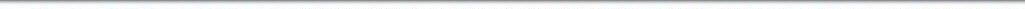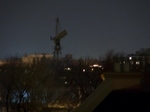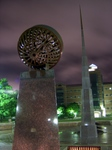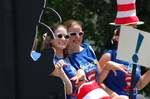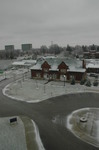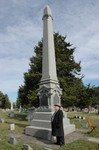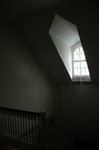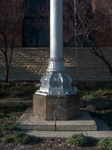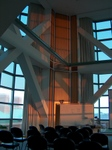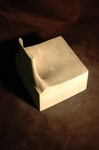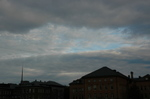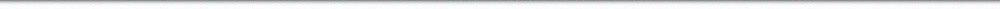N A V I G A T I O N D I G I T A L L I B R A R Y

## Repository: UIHistories Project: Book - Century of Physics (1973) [PAGE 20]

Caption: Book - Century of Physics (1973)
This is a reduced-resolution page image for fast online browsing.

< Previous Page [Displaying Page 20 of 39] Next Page >
[VIEW ALL PAGE THUMBNAILS]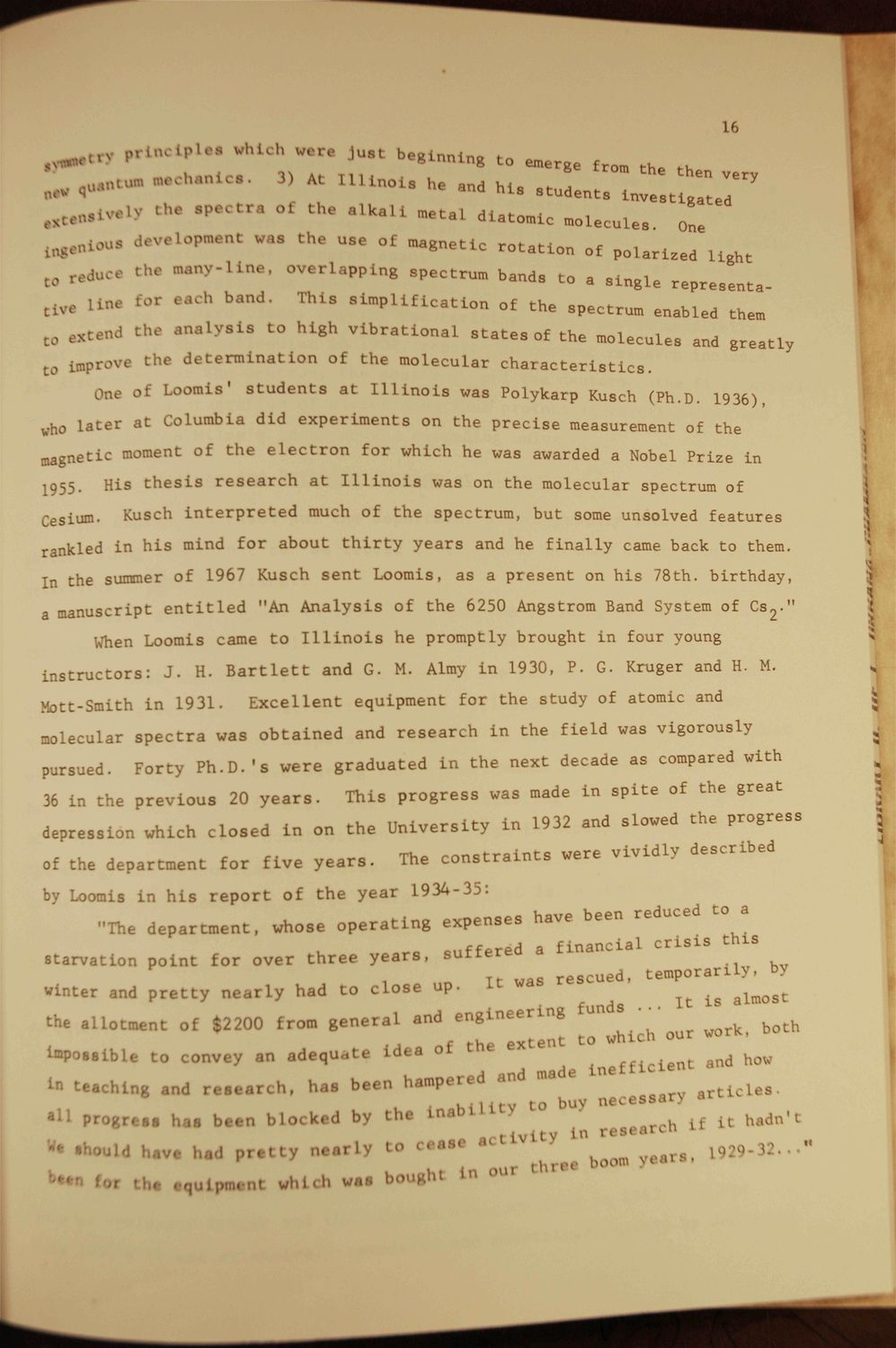### EXTRACTED TEXT FROM PAGE:

16

t r

, p r i n c i p l e . whKh were j u s t b e g i n n i n g t o emerge - o h . * •« P .. 3) A t m t „ o i a he

and h i 8

from

,. ^ ^

„ ^ ^ lighc T

;

qu.„mim

stents ^

rsten.ivel3

" ' •« * • alkali

B e t a l

diatomlc

^ i O U . d « V . l o p - n t was t h e u s e of m a g n e t i c r o t a t i o n of p o l a r i z e d

'

, J«c« t h e many-I i n , , l i n e for each b a n d .

o v e r l a p p i n g spectrum bands t o a s i n g l e r e p r e s e n t a T h i s s i m p l i f i c a t i o n of t h e spectrum enabled them characteristics.

Lve

0 extena t0

t h e a n a l y s i s t o h i g h v i b r a t i o n a l s t a t e s of t h e molecules and g r e a t l y

improve t h e d e t e r m i n a t i o n of t h e m o l e c u l a r

One of Loomis' s t u d e n t s a t I l l i n o i s was Polykarp Kusch (Ph.D. 1936),

l0

l a t e r a t Columbia d i d e x p e r i m e n t s on t h e p r e c i s e measurement of the j I

1

magnetic moment o f t h e e l e c t r o n f o r which he was awarded a Nobel P r i z e i n 1955. >sium. His t h e s i s r e s e a r c h a t I l l i n o i s was on t h e m o l e c u l a r spectrum of Kusch i n t e r p r e t e d much of t h e s p e c t r u m , b u t some unsolved f e a t u r e s

I

\

rankled i n h i s mind f o r a b o u t t h i r t y y e a r s and he f i n a l l y came back t o them. In the summer o f 1967 Kusch s e n t Loomis, a s a p r e s e n t on h i s 78th. b i r t h d a y , a manuscript e n t i t l e d "An A n a l y s i s of t h e 6250 Angstrom Band System of C s 2 - "

1 5

When Loomis came t o I l l i n o i s h e p r o m p t l y b r o u g h t i n four young i n s t r u c t o r s : J . H. B a r t l e t t Mott-Smith i n 1 9 3 1 . and G. M. Almy i n 1930, P . G. Kruger and H. M. e q u i p m e n t for t h e s t u d y of atomic and

Excellent

molecular s p e c t r a was o b t a i n e d and r e s e a r c h i n t h e f i e l d was v i g o r o u s l y pursued. F o r t y P h . D . ' s w e r e g r a d u a t e d i n t h e n e x t decade a s compared with T h i s p r o g r e s s was made i n s p i t e of the g r e a t

* *

36 in the p r e v i o u s 20 y e a r s . depression which c l o s e d of the d e p a r t m e n t

5 |

i n on t h e U n i v e r s i t y i n 1932 and slowed the p r o g r e s s The c o n s t r a i n t s were v i v i d l y d e s c r i b e d 1934-35: ^ ^ ' J ^

f o r five y e a r s .

by Loomis i n h i s r e p o r t o f t h e y e a r "The d e p a r t m e n t ,

whose o p e r a t i n g e x p e n s e s have

starvation point for over t h r e e y e a r s , suffered a financia „ I t was r e s c u e d , t e m p o r a r i l y , by winter and p r e t t y n e a r l y had t o c l o s e u p . Li wa ^ ^ ^ the a n o i n t of \$2200 f r o . g e n e r a l and * ~ ^ ^ nu h our work, both and how

t l c l M

impossible t o convey a n adequ... i d e a of l» teang and r e s e a r c h , h a s b e e n hampe.

Lnefficlent

• u, i uv to buv necessary an.

^